# [Free] Age calculator Extension

Hello koder , this is my age calculator Extension created by Mr_koder#### Documentation

1. Calculate Exact Age Block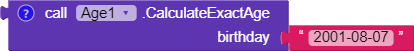• Description: This block calculates the exact age of a person in years, months, and days based on the given birthday.
• Input: A string representing the person’s birthday in the format ‘yyyy-MM-dd’.
• Output: A YailList containing three elements: [`years`, `months`, `days`], where ‘years’ is the age in years, ‘months’ is the remaining months, and ‘days’ is the remaining days."

1. Calculate Age In Years Block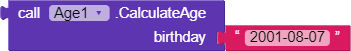• Description: This block calculates the age of a person in years based on the given birthday.
• Input: A string representing the person’s birthday in the format “yyyy-MM-dd”
• Output: An integer representing the person’s age in years.

1. Calculate Age in Days Block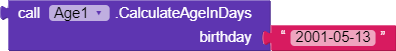• Description: This block calculates the age of a person in days based on the given birthday.
• Input: A string representing the person’s birthday in the format “yyyy-MM-dd”
• Output: An integer representing the person’s age in days.

1. Calculate Age in Months Block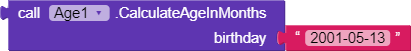• Description: This block calculates the age of a person in months based on the given birthday.
• Input: A string representing the person’s birthday in the format “yyyy-MM-dd”
• Output: An integer representing the person’s age in months.

1. Is Birthday Today Block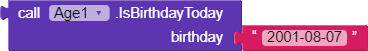• Description: This block checks if today is the person’s birthday based on the given birthday.
• Input: A string representing the person’s birthday in the format “yyyy-MM-dd”
• Output: A boolean value, `true` if today is the person’s birthday, and `false` otherwise.

### Explanation

this extension compares the device’s current date with the provided birthday to calculate the person’s exact age in years, months, and days.

com.age.calculate.mrkoder.aix (7.3 KB)

Thanks .

Mr_koder

5 Likes

Taifun

1 Like

OK edited in the extension file
thanks @Taifun

I think, This will return age only in years,

You can add a function to get exact age in years, months and days.

2 Likes